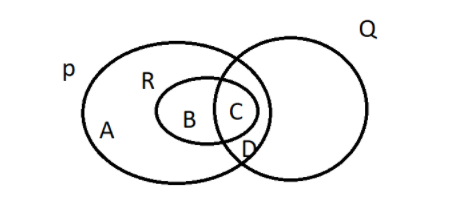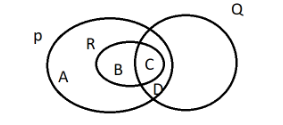Courses
Courses for Kids
Free study material
Offline Centres
MoreLast updated date: 06th Dec 2023
Total views: 279.9k
Views today: 4.79k

# In the venn diagram , the universal set , $\xi = P \cup Q \cup R$ .Which of the four regions labelled A, B, C and D represents the sets $P \cap Q \cap R$ ?Verified
279.9k+ views
Hint: In order to solve the given question , we should know the three important concepts related to the question that is Venn diagram , Sets – concept of union and intersection . A venn diagram shows us the relation between or among a finite group of sets . Consider we have 2 sets – X and Y . The Union of these two sets X and Y is any region which includes the elements of either X or Y. The Intersection of these two sets X and Y is any region which includes the elements of both X and Y.

Let us consider an example similar to the question to understand union and intersection of sets .
Consider the two sets X and Y having their elements as given : $X = \left\{ {1,3,5} \right\}$ and $Y = \left\{ {1,2,4,5} \right\}$ .
Union of set is depicted by the symbol ‘ $\cup$ ’ , which tells the that the two sets which we have taken X and Y is equivalent to the set of elements , which are considered in set X , in set Y or in both the sets X and Y . the union of two sets will be –
$X \cup Y = \{ 1,2,3,4,5\}$
Intersection of sets is depicted by the symbol ‘ $\cap$ ’ , which tells them that the two sets which we have taken X and Y are equivalent to the set of elements , which are common to set X and set Y both . The intersection of two sets will be –
$X \cap Y =\{ 1,5\}$ .
Now you have good knowledge of union and intersection so we can solve similarly the given question .
We have to calculate the intersection of 3 sets $P \cap Q \cap R$ that means we have to find the region which is common to the regions of these 3 sets P, Q, R .We can see in the image the following result –
Region A includes only set P .
Region B includes only set P and set R , here Q is missing .
Region D includes only set P and set Q , here R is missing .
Region C represents all the regions with the sets $P \cap Q \cap R$ .

Note: Always try to understand the mathematical statement carefully and keep things distinct .
Remember the properties and apply appropriately .
Try to solve the question using the venn diagram which makes it visual and easy to understand .
Choose the options wisely , it's better to break the question and then solve part by part .
Cross check the answer and always keep the final answer simplified .